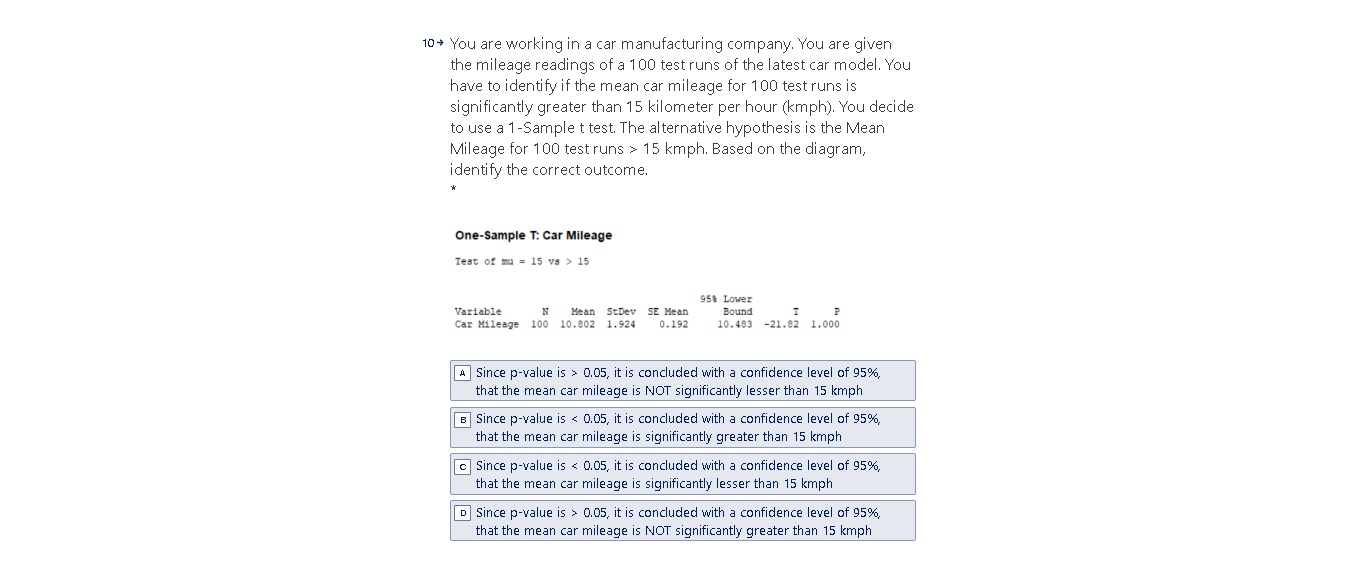# Question Solved1 Answer10+ You are working in a car manufacturing company. You are given the mileage readings of a 100 test runs of the latest car model. You have to identify if the mean car mileage for 100 test runs is significantly greater than 15 kilometer per hour (kmph). You decide to use a 1 -Sample t test. The alternative hypothesis is the Mean Mileage for 100 test runs > 15 kmph. Based on the diagram, identify the correct outcome. One-Sample T: Car Mileage Test of mu = 15 vs > 15 Variable N Mean StDev SE Mean Car Mileage 100 10.802 1.924 0.192 95% Lower Bound 10.483 -21.82 1.000 A Since p-value is > 0.05, it is concluded with a confidence level of 95%, that the mean car mileage is NOT significantly lesser than 15 kmph B Since p-value is < 0.05, it is concluded with a confidence level of 95%, that the mean car mileage is significantly greater than 15 kmph Since p-value is < 0.05, it is concluded with a confidence level of 95%, that the mean car mileage is significantly lesser than 15 kmph D Since p-value is > 0.05, it is concluded with a confidence level of 95%, that the mean car mileage is NOT significantly greater than 15 kmphMLHL0W The Asker · Probability and StatisticsTranscribed Image Text: 10+ You are working in a car manufacturing company. You are given the mileage readings of a 100 test runs of the latest car model. You have to identify if the mean car mileage for 100 test runs is significantly greater than 15 kilometer per hour (kmph). You decide to use a 1 -Sample t test. The alternative hypothesis is the Mean Mileage for 100 test runs > 15 kmph. Based on the diagram, identify the correct outcome. One-Sample T: Car Mileage Test of mu = 15 vs > 15 Variable N Mean StDev SE Mean Car Mileage 100 10.802 1.924 0.192 95% Lower Bound 10.483 -21.82 1.000 A Since p-value is > 0.05, it is concluded with a confidence level of 95%, that the mean car mileage is NOT significantly lesser than 15 kmph B Since p-value is < 0.05, it is concluded with a confidence level of 95%, that the mean car mileage is significantly greater than 15 kmph Since p-value is < 0.05, it is concluded with a confidence level of 95%, that the mean car mileage is significantly lesser than 15 kmph D Since p-value is > 0.05, it is concluded with a confidence level of 95%, that the mean car mileage is NOT significantly greater than 15 kmph
More
Transcribed Image Text: 10+ You are working in a car manufacturing company. You are given the mileage readings of a 100 test runs of the latest car model. You have to identify if the mean car mileage for 100 test runs is significantly greater than 15 kilometer per hour (kmph). You decide to use a 1 -Sample t test. The alternative hypothesis is the Mean Mileage for 100 test runs > 15 kmph. Based on the diagram, identify the correct outcome. One-Sample T: Car Mileage Test of mu = 15 vs > 15 Variable N Mean StDev SE Mean Car Mileage 100 10.802 1.924 0.192 95% Lower Bound 10.483 -21.82 1.000 A Since p-value is > 0.05, it is concluded with a confidence level of 95%, that the mean car mileage is NOT significantly lesser than 15 kmph B Since p-value is < 0.05, it is concluded with a confidence level of 95%, that the mean car mileage is significantly greater than 15 kmph Since p-value is < 0.05, it is concluded with a confidence level of 95%, that the mean car mileage is significantly lesser than 15 kmph D Since p-value is > 0.05, it is concluded with a confidence level of 95%, that the mean car mileage is NOT significantly greater than 15 kmph